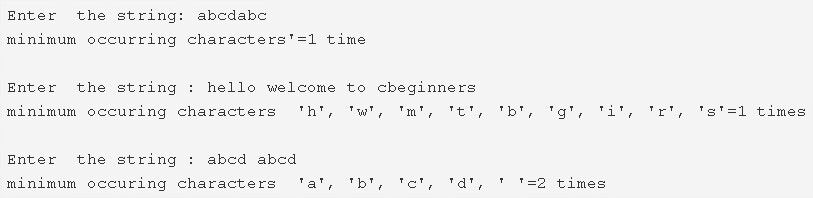# C Program To Find Lowest Frequency Character In A String | C Programs

C program to find the lowest frequency character in a string – In this article, we will brief in on the several ways to find the highest frequency character in a string in C programming.

Suitable examples and sample programs have also been added so that you can understand the whole thing very clearly. The compiler has also been added with which you can execute it yourself.

The means used in this distinct piece are as follows:

• Using Standard Method
• Using Function

A string is nothing but an array of characters. The value of a string is determined by the terminating character. Its value is considered to be 0.As you can see in the image uploaded above, firstly, you need to enter the string of your concern.

Let’s take the first example itself.

The string entered is abcdabc

As we can see, the least frequency is of the character ‘d’: Only once.

Hence, multiple ways to do the same in C programming are as follows:

## Using Standard Method

1. Initialize count=0, declare the character array s and int array a.

2) The for loop iterates through the string until the last element of the string becomes null. The value of j at the last iteration of for loop indicates the length of the string.

3) Initialize the j value to the variable k and variable n.

4) The outer for loop iterates through the string from i=0 to i<length of the string.

a) Initialize a[i]=length of the string and count=1.

b) The inner for loop compares s[i] with remaining elements of the string. If s[i] matches then increase the count value and initialize the element with null where it appears repeatedly. store the count value at a[i].

c) Store the count value at a[i].

If count value <k then initialize k=count.

5) The for loop iterates with the structure for(j=0;j<;j++) through the integer array which contains count values of the characters of the string.

If the count value a[j] equal to the minimum count value k then print the element s[j] and the k value.

Output:

### Using Function

1. The function stringlength(char *s) returns the string length.

2) The main() function calls the printmin(char *s) by passing the string as an argument to the function.

3)The function printmin(char *s) prints the minimum frequency elements.

a) The outer for loop iterates through the string until the last character of the string becomes to null.

b) The inner for loop finds the frequency of each character of the string and place the frequency numbers into the integer array a[].

c) If the frequency of any character is equal to minimum frequency k then print that element and print the k value.

Output:
x

## C Program To Print Number Of Days In A Month | Java Tutoring

C program to input the month number and print the number of days in that ...